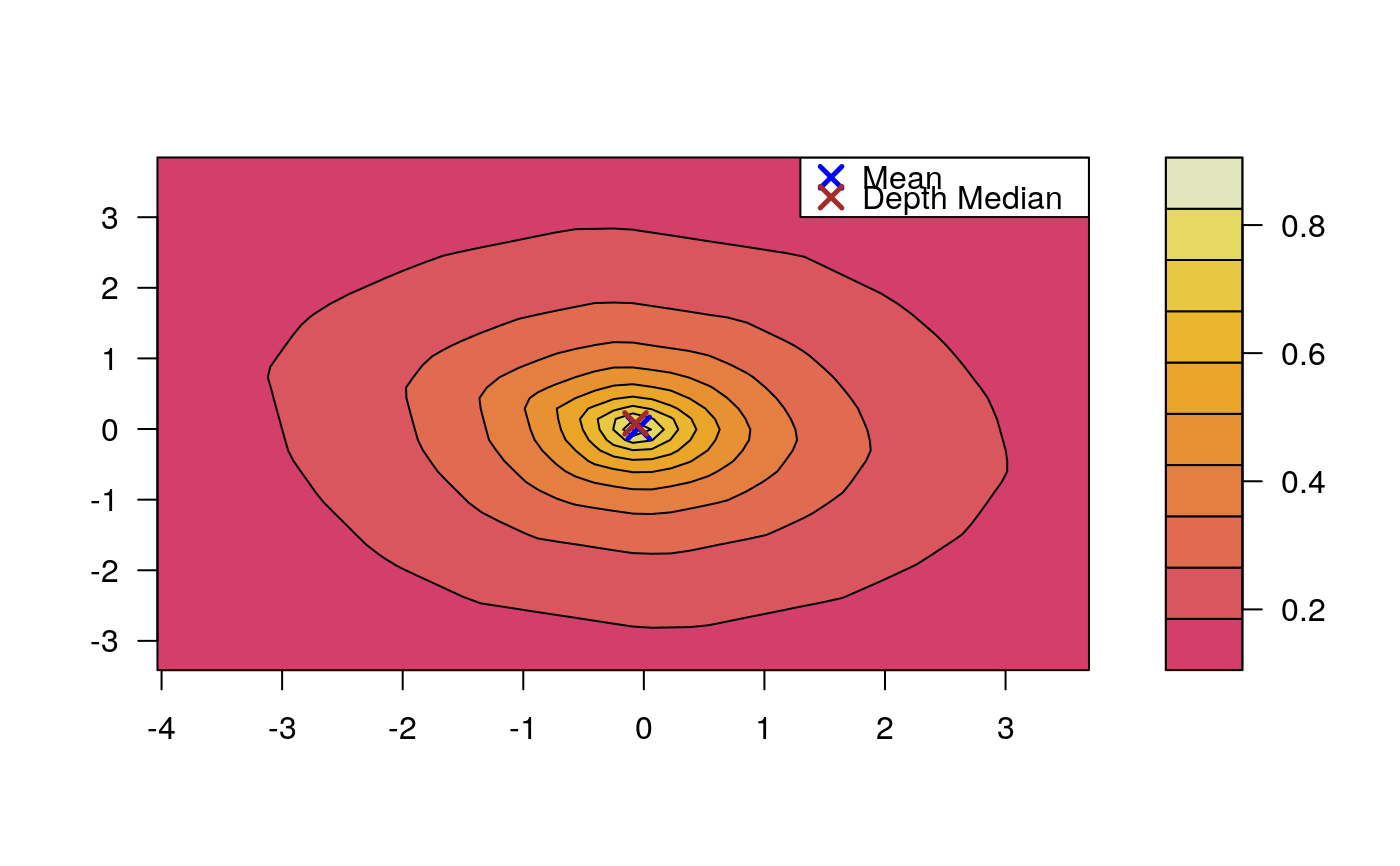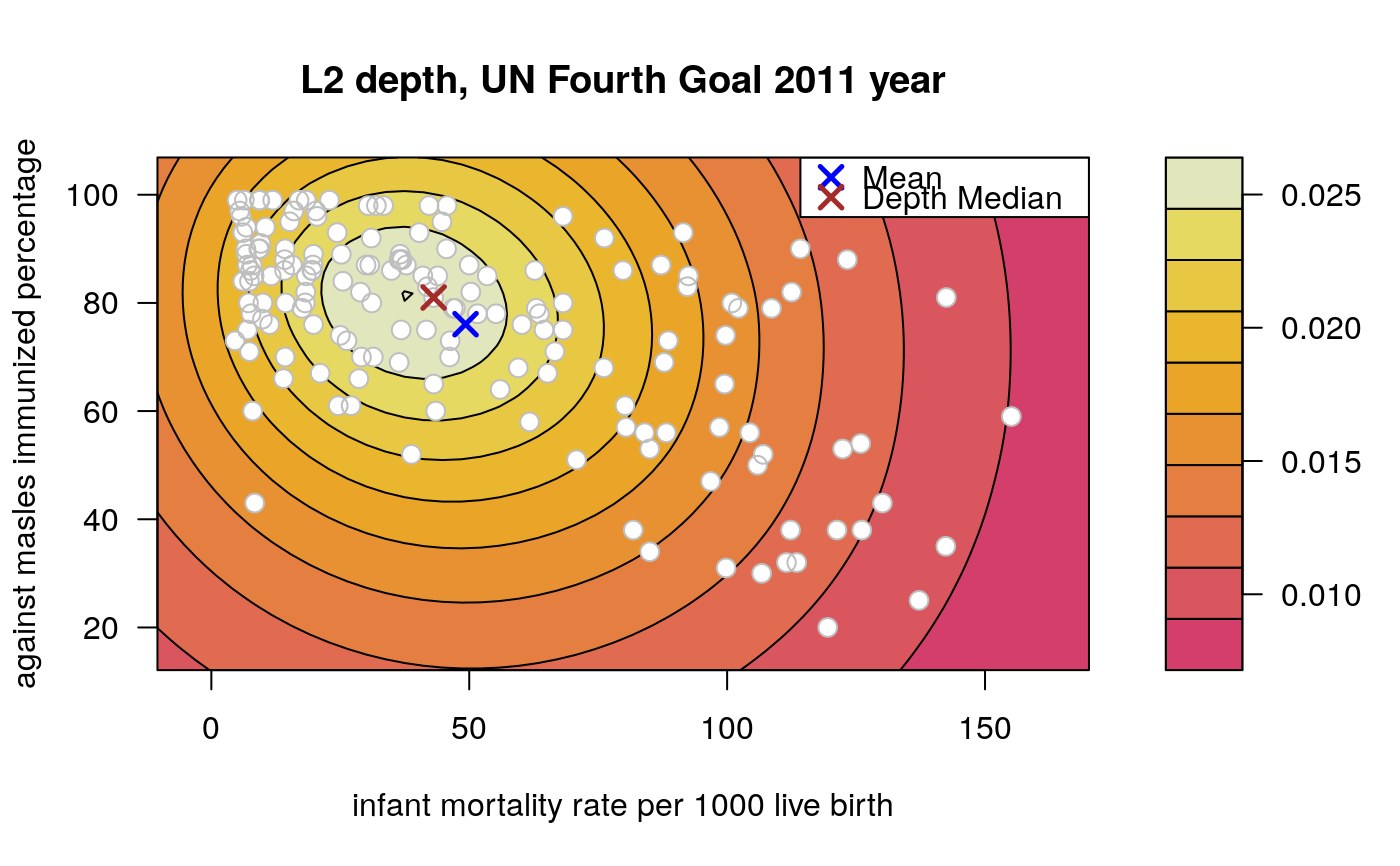Draws an approximate contours of depth for bivariate data.

depthContour(
x,
xlim = extendrange(x[, 1], f = 0.1),
ylim = extendrange(x[, 2], f = 0.1),
n = 50,
pmean = TRUE,
mcol = "blue",
pdmedian = TRUE,
mecol = "brown",
legend = TRUE,
points = FALSE,
colors = heat_hcl,
levels = 10,
depth_params = list(),
graph_params = list(),
contour_method = c("auto", "convexhull", "contour")
)

## Arguments

x Bivariate data Determines the width of x-axis. Determines the width of y-axis. Number of points in each coordinate direction to be used in contour plot. Logical. If TRUE mean will be marked. Determines the color of lines describing the mean. Logical. If TRUE depth median will be marked. Determines the color of lines describing the depth median. Logical. If TRUE legend for mean and depth median will be drawn. Logical. If TRUE points from matrix x will be drawn. function for colors pallete (e.g. gray.colors). number of levels for color scale. list of parameters for function depth (method, threads, ndir, la, lb, pdim, mean, cov, exact). list of graphical parameters for functions filled.contour and contour (e.g. lwd, lty, main). determines the method used to draw the contour lines. The default value ("auto") tries to determine the best method for given depth function. "convexhull" uses a convex hull algorithm to determine boundaries. "contour" uses the algorithm from filled.contour.

## Details

The set of all points that have depth at least $$\alpha$$ is called $$\alpha$$-trimmed region. The $$\alpha$$-trimmed region w.r.t. $$F$$ is denoted by $${D}_{\alpha}(F)$$, i.e., $${D}_{\alpha}(F) = \left\{ z \in {{{R}} ^ {d}}:D(z, F) \ge \alpha\right\}.$$

depthPersp

## Examples

# EXAMPLE 1
set.seed(123)
x <- mvrnorm(1000, c(0, 0), diag(2))
depthContour(x, colors = gray.colors)# with points
depthContour(x, points = TRUE)depthContour(x, points = FALSE, levels = 10)# EXAMPLE 2
data(inf.mort, maesles.imm)
data1990 <- na.omit(cbind(inf.mort[, 1], maesles.imm[, 1]))
depthContour(data1990, n = 50, pmean = TRUE, mcol = "blue",
pdmedian = TRUE, mecol = "brown", legend = TRUE, points = TRUE,
depth_params = list(method = "LP"),
graph_params = list(
xlab = "infant mortality rate per 1000 live birth",
ylab = "against masles immunized percentage",
main = "L2 depth, UN Fourth Goal 2011 year"))#EXAMPLE 3
data("france")
depthContour(france,
depth_params = list(method = "Tukey"),
points = TRUE
)